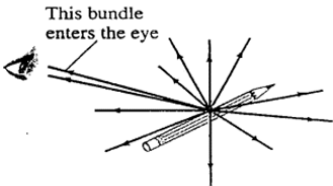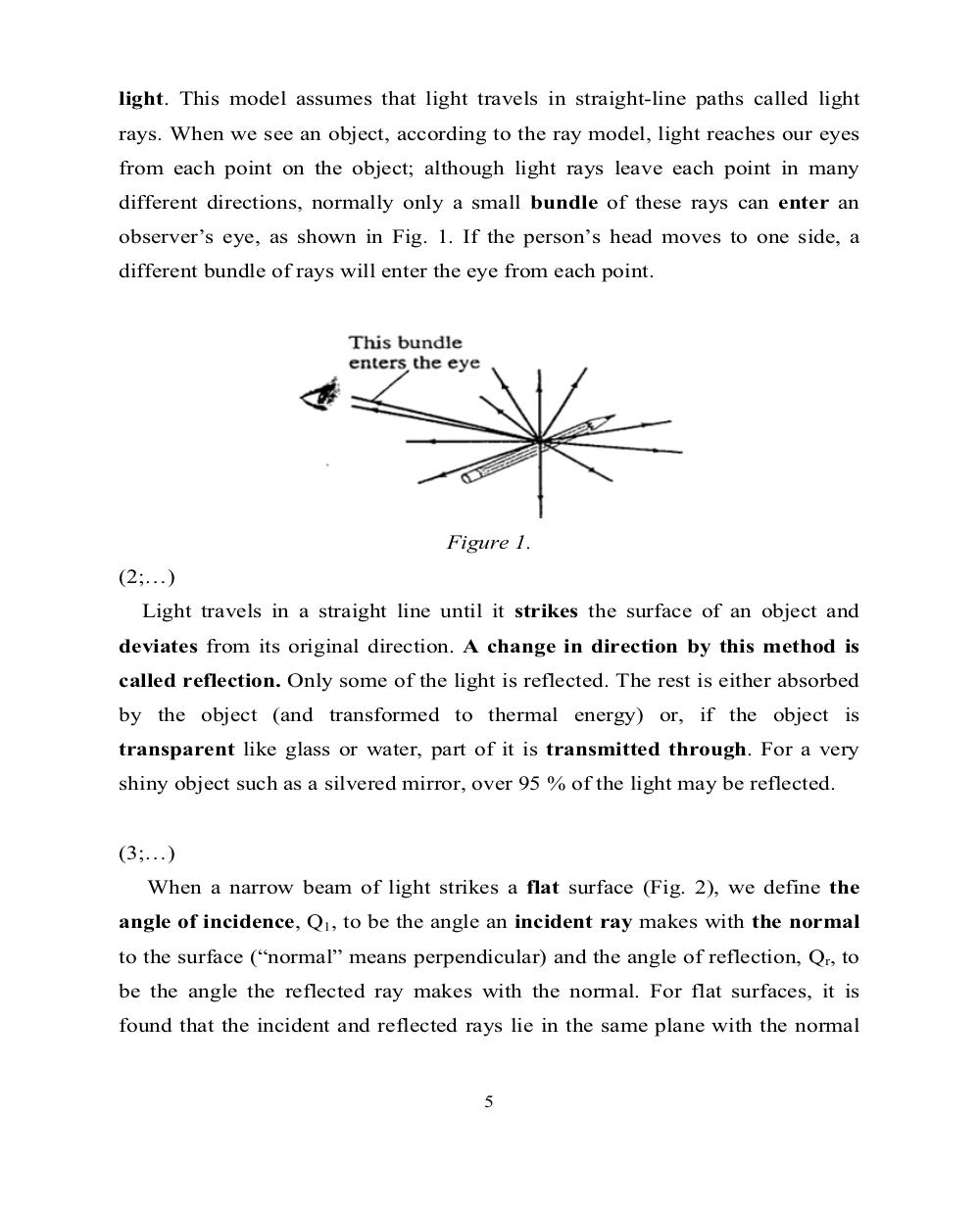# Английский язык. Ч.3 (Units 9,10). Ильичева Н.А - 5 стр.

Составители:

Рубрика:

• ## Иностранный языкlight. This model assumes that light travels in straight-line paths called light
rays. When we see an object, according to the ray model, light reaches our eyes
from each point on the object; although light rays leave each point in many
different directions, normally only a small bundle of these rays can enter an
observer’s eye, as shown in Fig. 1. If the person’s head moves to one side, a
different bundle of rays will enter the eye from each point.
Figure 1.
(2;…)
Light travels in a straight line until it strikes the surface of an object and
deviates from its original direction. A change in direction by this method is
called reflection. Only some of the light is reflected. The rest is either absorbed
by the object (and transformed to thermal energy) or, if the object is
transparent like glass or water, part of it is transmitted through. For a very
shiny object such as a silvered mirror, over 95 % of the light may be reflected.
(3;…)
When a narrow beam of light strikes a flat surface (Fig. 2), we define the
angle of incidence, Q
1
, to be the angle an incident ray makes with the normal
to the surface (“normal” means perpendicular) and the angle of reflection, Q
r
, to
be the angle the reflected ray makes with the normal. For flat surfaces, it is
found that the incident and reflected rays lie in the same plane with the normal
5light. This model assumes that light travels in straight-line paths called light
rays. When we see an object, according to the ray model, light reaches our eyes
from each point on the object; although light rays leave each point in many
different directions, normally only a small bundle of these rays can enter an
observer’s eye, as shown in Fig. 1. If the person’s head moves to one side, a
different bundle of rays will enter the eye from each point.

Figure 1.
(2;…)
Light travels in a straight line until it strikes the surface of an object and
deviates from its original direction. A change in direction by this method is
called reflection. Only some of the light is reflected. The rest is either absorbed
by the object (and transformed to thermal energy) or, if the object is
transparent like glass or water, part of it is transmitted through. For a very
shiny object such as a silvered mirror, over 95 % of the light may be reflected.

(3;…)
When a narrow beam of light strikes a flat surface (Fig. 2), we define the
angle of incidence, Q1, to be the angle an incident ray makes with the normal
to the surface (“normal” means perpendicular) and the angle of reflection, Qr, to
be the angle the reflected ray makes with the normal. For flat surfaces, it is
found that the incident and reflected rays lie in the same plane with the normal

5